出租广告位,需要合作请联系站长

+关注

# 什么是opencv?

1. OpenCV（Open Source Computer Vision Library）是开源的计算机视觉和机器学习库，提供了C++、C以及python等接口，并支持Windows、Linux、Android、MacOS平台。
2. 在2016年以后，深度学习的应用越来越广泛，OpenCV里也添加了CNN之类的模块，可以与Tensorflow、Caffe2这些框架训练出来的模型对接。

## 图像数字化基础知识508行（高度）672列（宽度）的二维数字矩阵。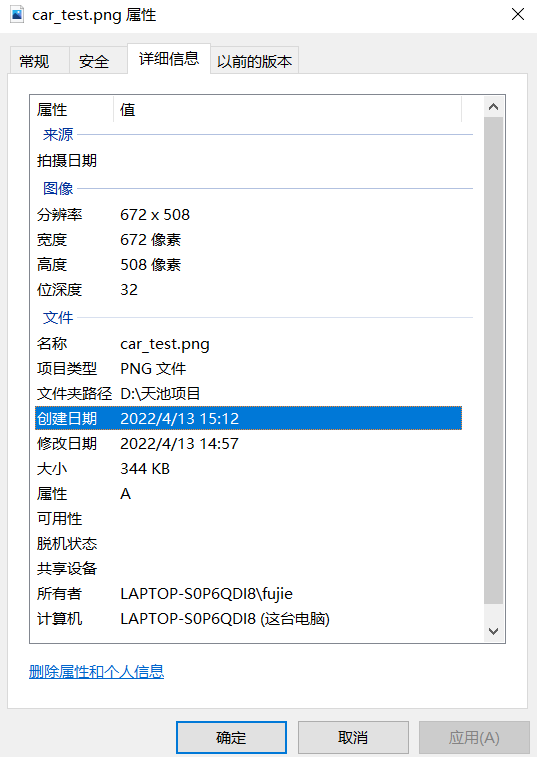## 图像获取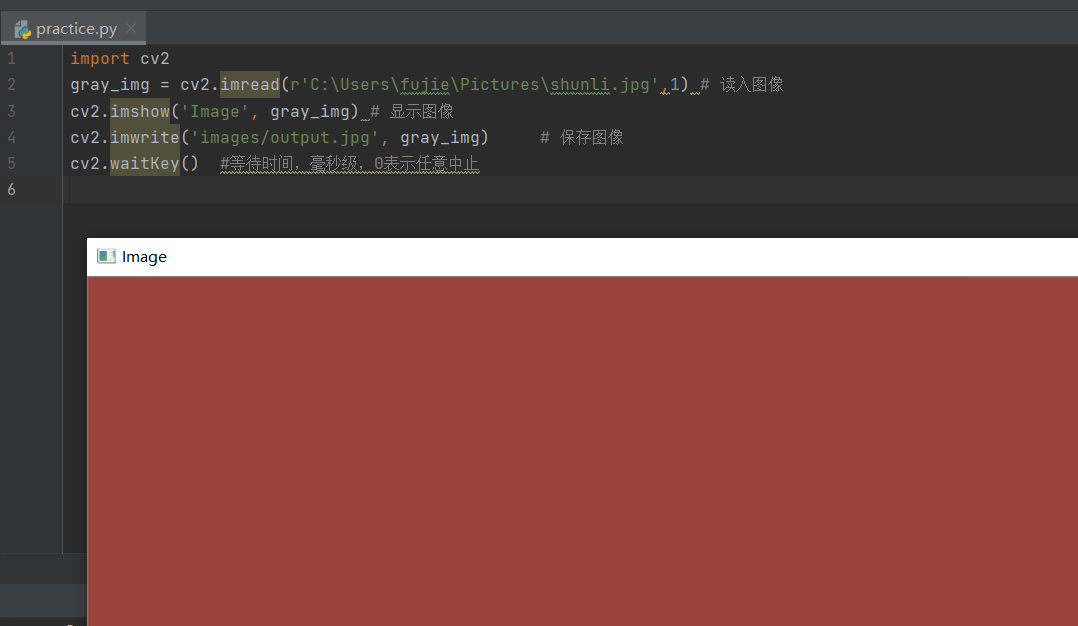``````import cv2
cv2.imshow('Image', gray_img) # 显示图像
cv2.imwrite('images/output.jpg', gray_img)     # 保存图像
cv2.waitKey()  #等待时间，毫秒级，0表示任意中止
``````

## 图像变换

• 图像增强的目的是要改善图像的视觉效果，针对给定图像的应用场合，有目的的增强图像的整体或局部特性，将原来不清晰的图像变得清晰或增强某些感兴趣的特征，扩大图像中不同物体的特征之间的差别，抑制不感兴趣的特征，使之改善图像质量、丰富信息量，将强图像判读和识别效果，满足某些特征分析的需求。

### 改变大小

（x,y,3)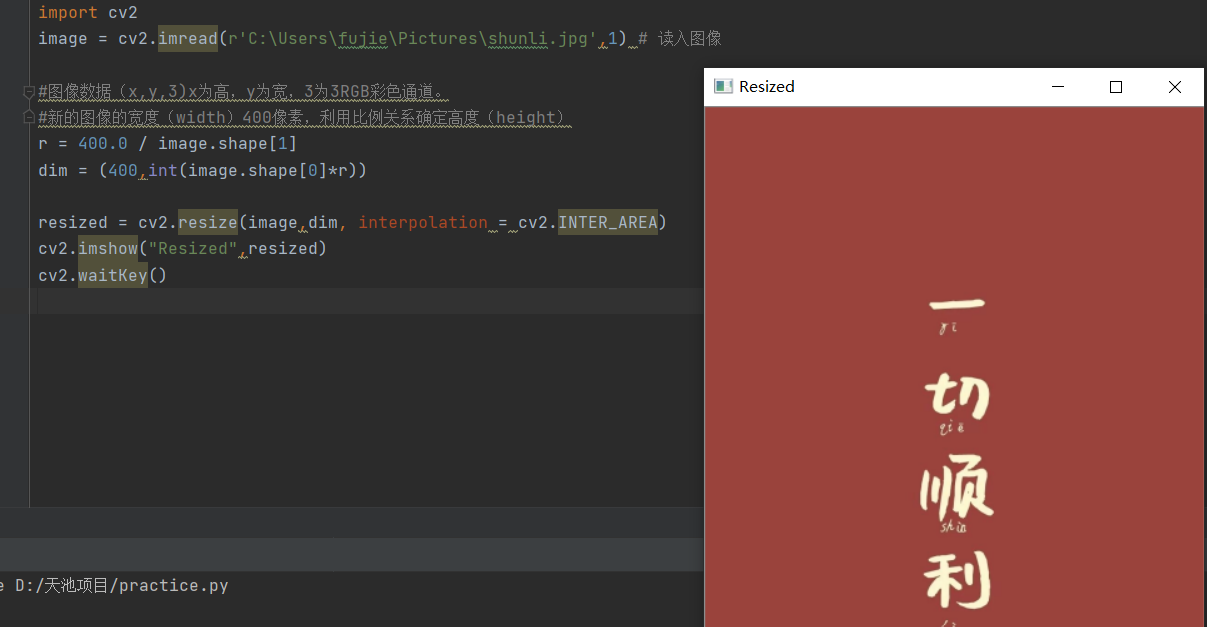# 车牌识别内容分析

1. 汽车牌照自动识别技术是把处理图像的方法与计算机的软件技术相连接在一起，以准确识别出车牌牌照的字符为目的，将识别出的数据传送至交通实时管理系统，以最终实现交通监管的功能。
2. 我国的汽车车牌一般由七个字符和一个点组成。
车牌识别大致分为四部分：图像获取（从视觉传感器中获取车辆的图像信息）——定位车牌（从原始图像中找到车牌的位置）——字符分割（将车牌进行分割，分割出七个字符用于后续的字符识别）——字符识别（对分离出的单个字符进行识别，从而识别出车牌的值）

## 定位车牌

1. 基于颜色的分割：利用颜色空间的信息，包括彩色边缘算法、颜色距离算法、相似度算法。
2. 基于纹理的分割：如利用车牌区域水平方向的纹理特征进行分割，包括小波纹理、水平梯度差纹理。
3. 基于边缘检测的分割
4. 基于数学形态法的分割

### 图像降噪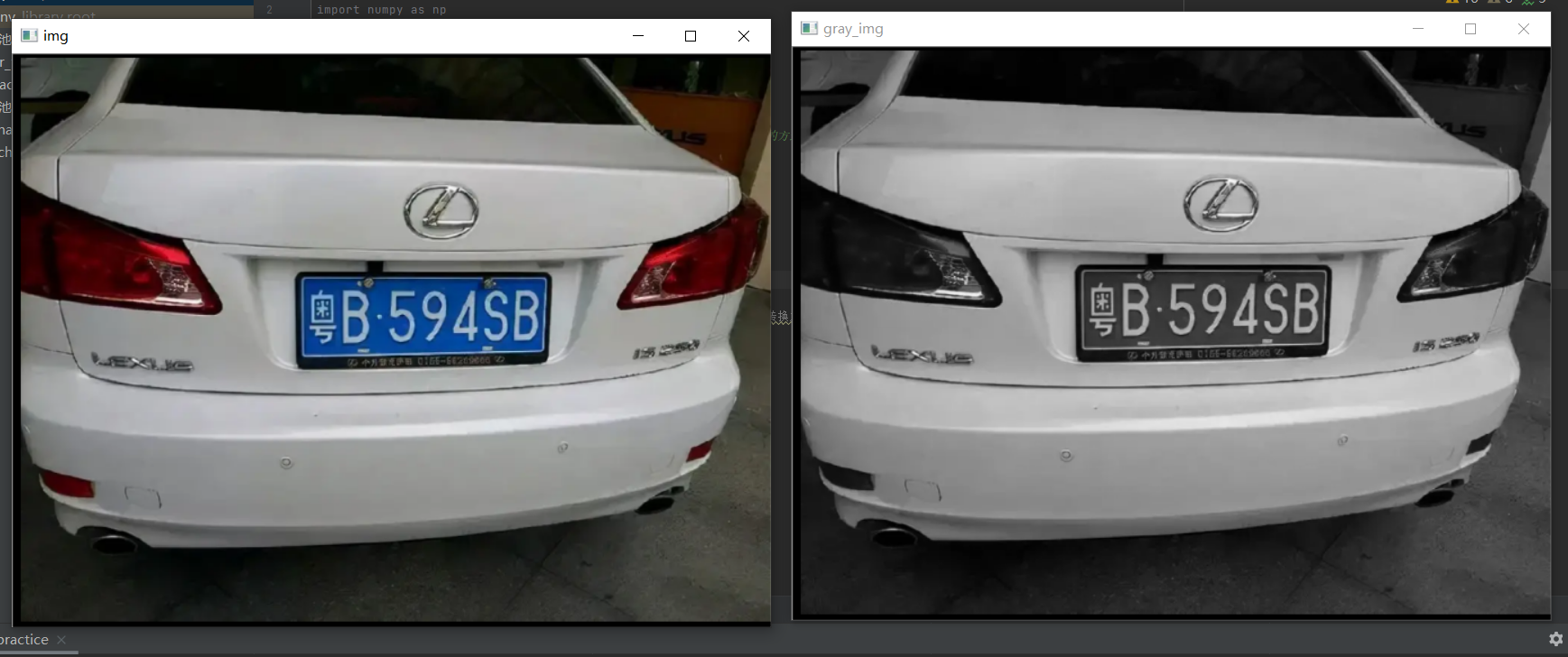——得到的图像与原始图像相比有些轻微的模糊。这样，单独的一个像素点在经过高斯平滑的图像上变得几乎没有影响。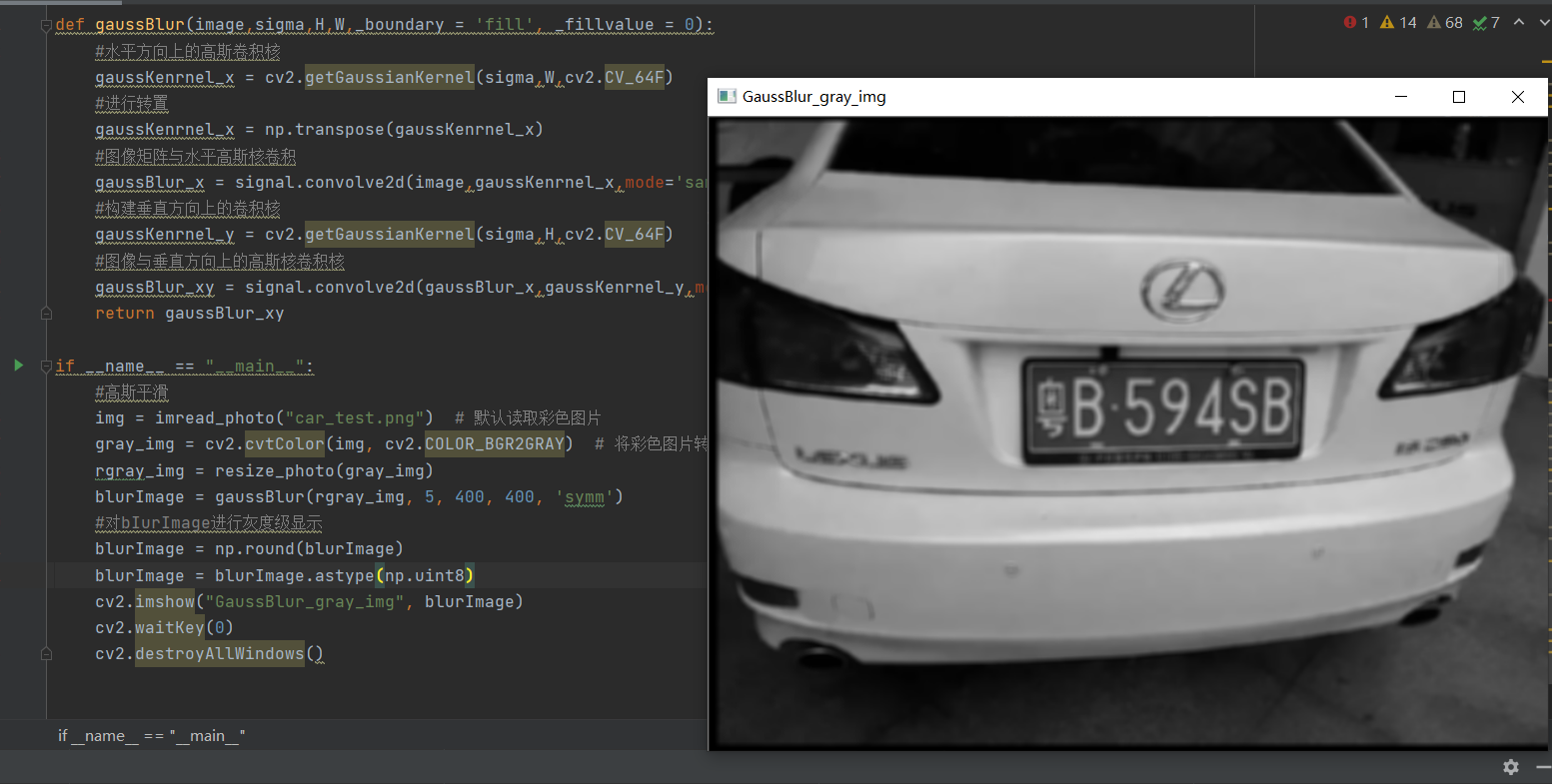• OpenCV之高斯平滑：
高斯平滑是通过做两个矩阵之间的二维离散卷积运算完成的，高斯卷积核和原始图像进行卷积。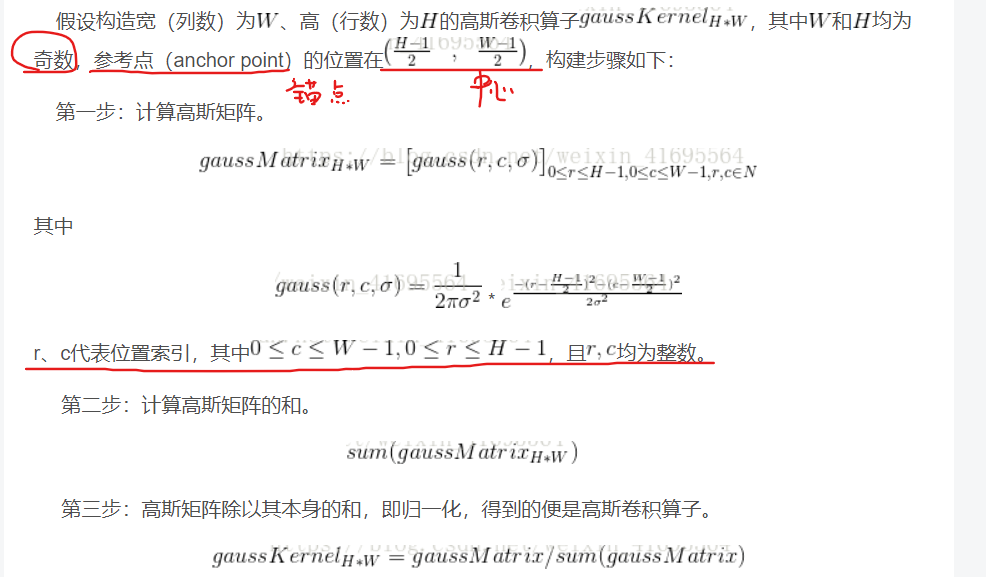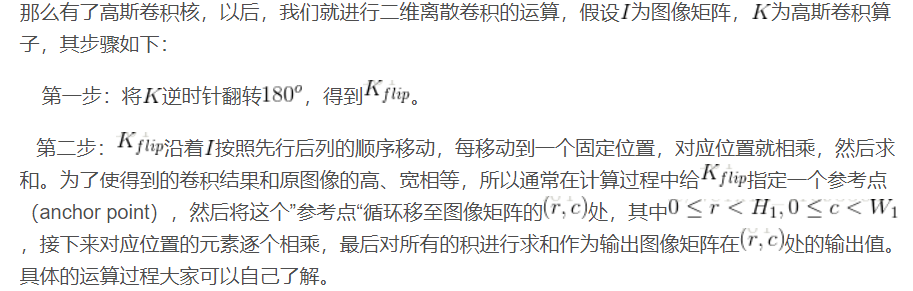• 卷积：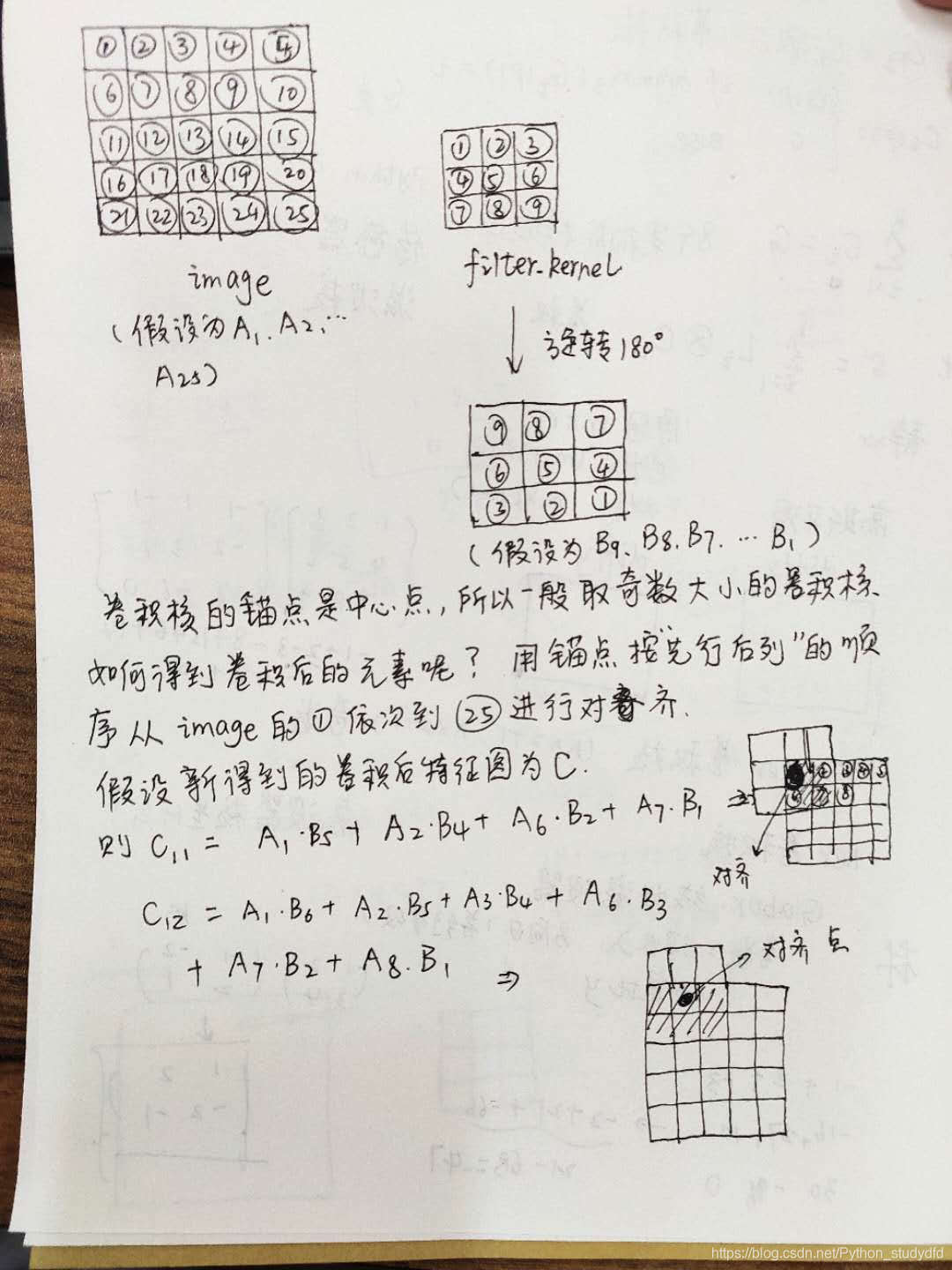• 在OpenCV中给出了构建一维垂直方向上的高斯卷积核的函数：Mat getGaussianKernel(int ksize, double sigma, in ktype = CV/_64F)

### 形态学处理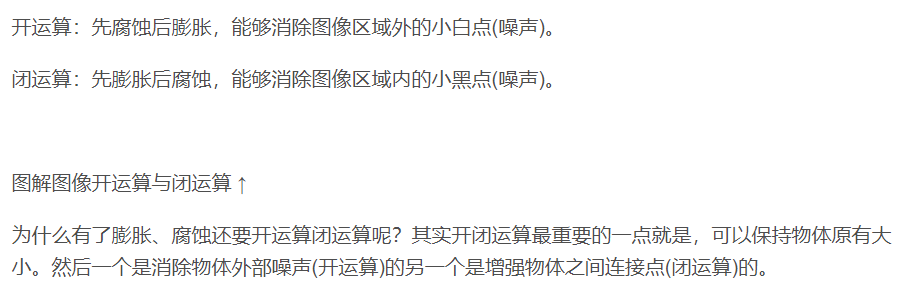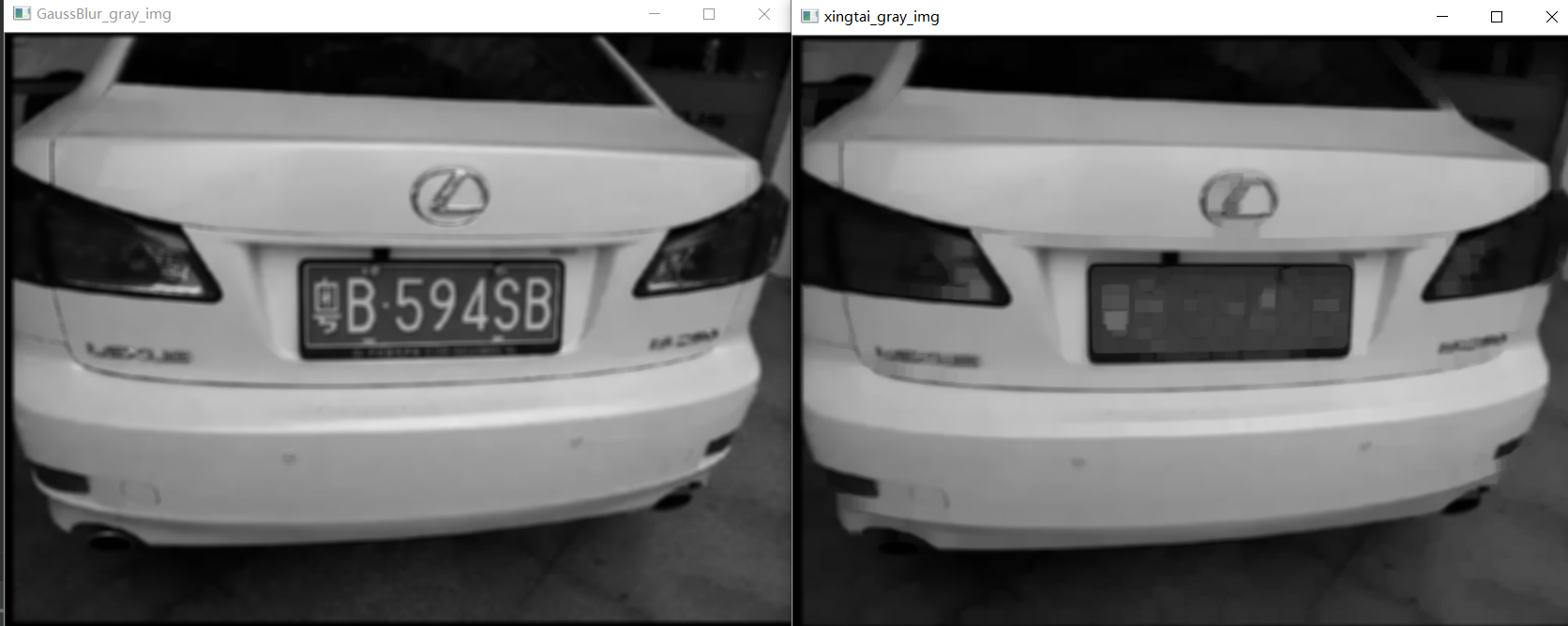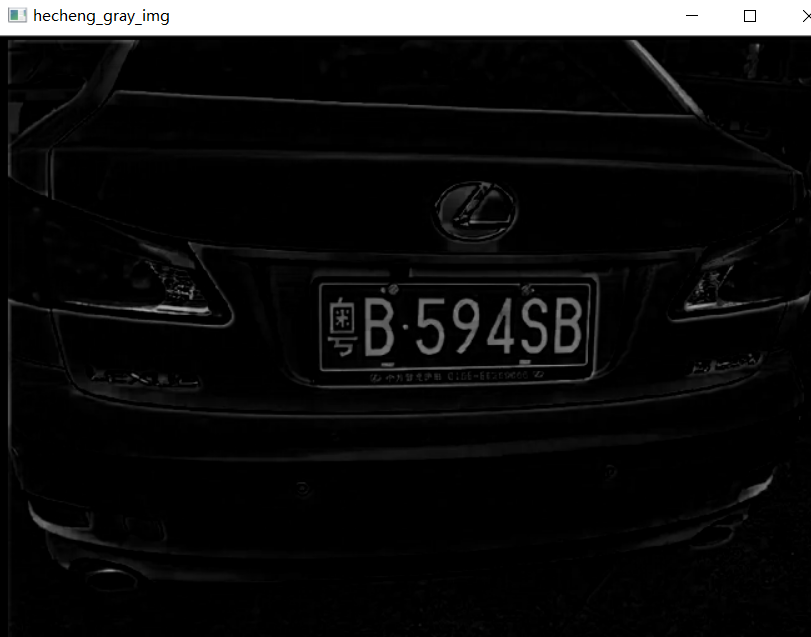### 阈值分割+边缘检测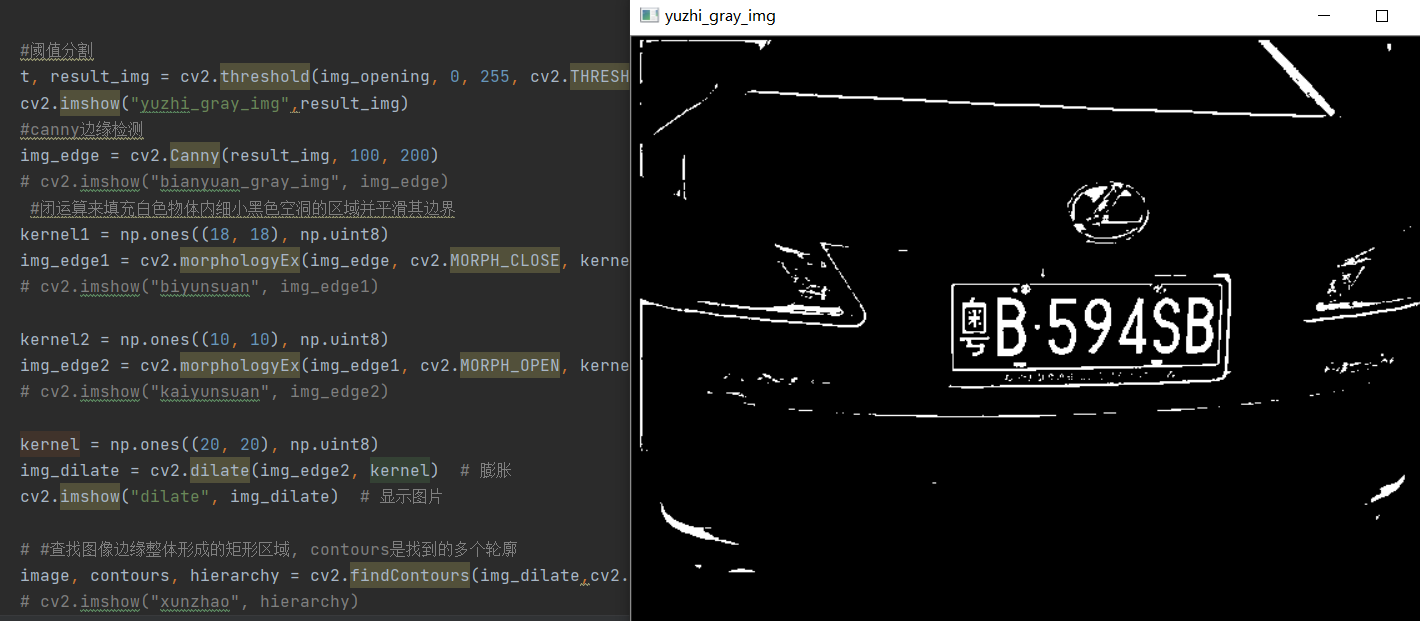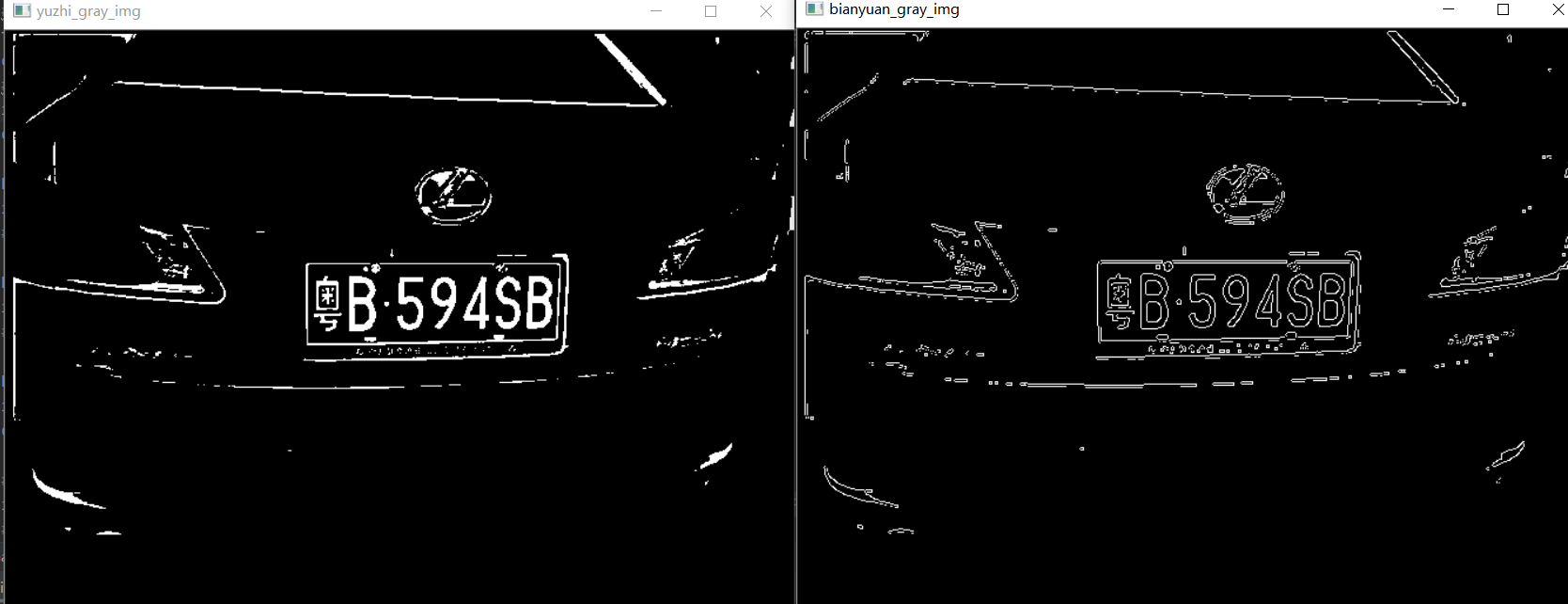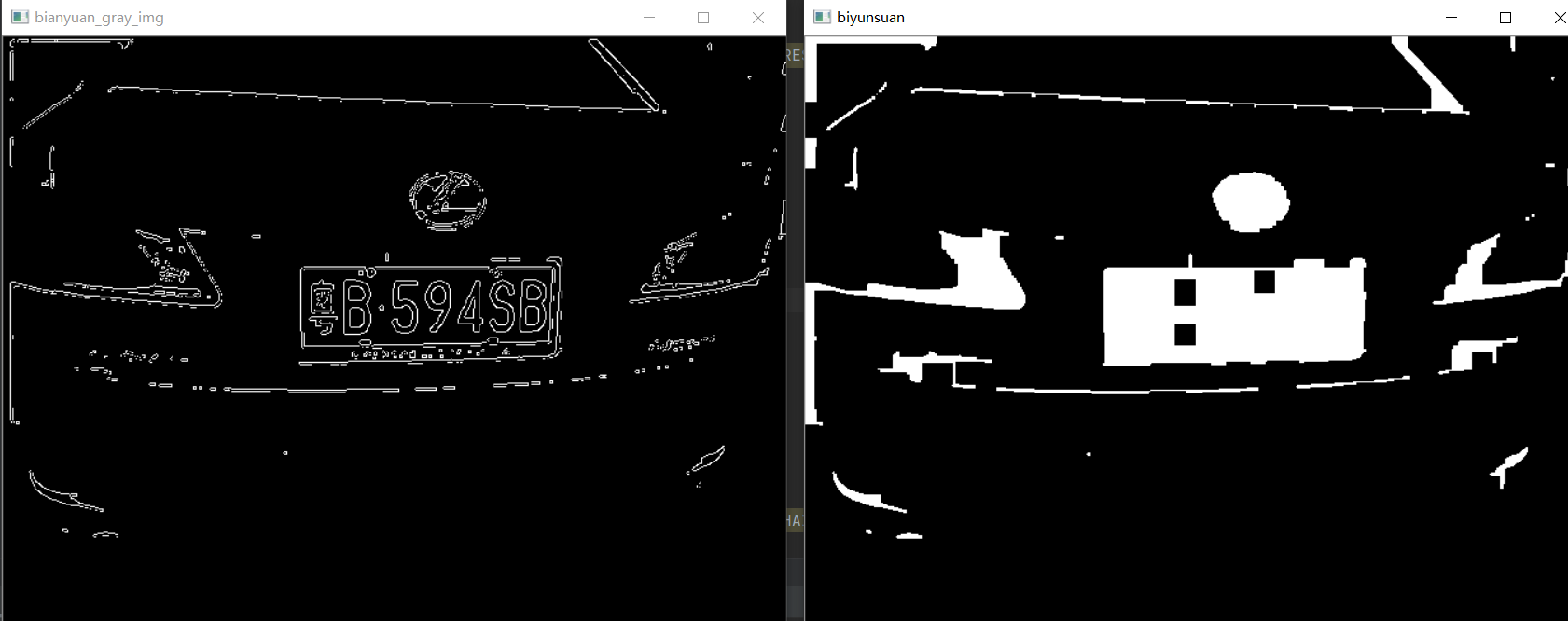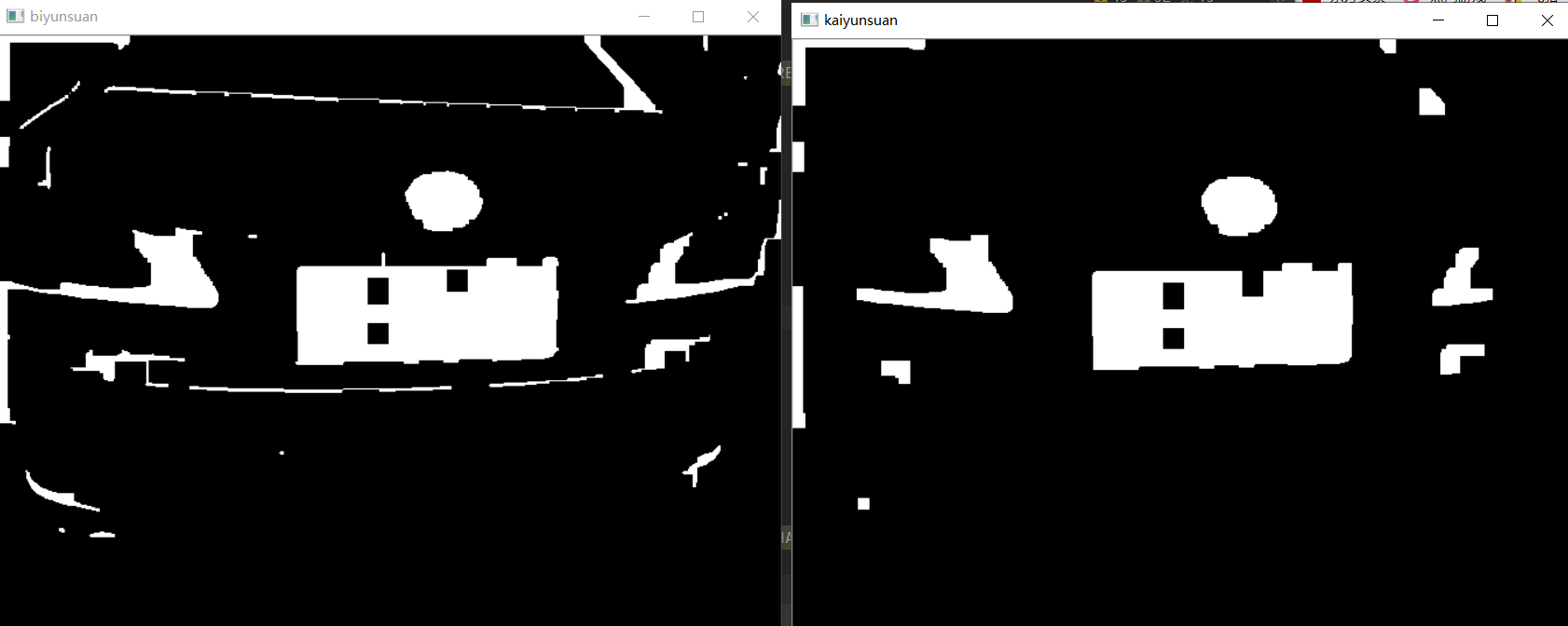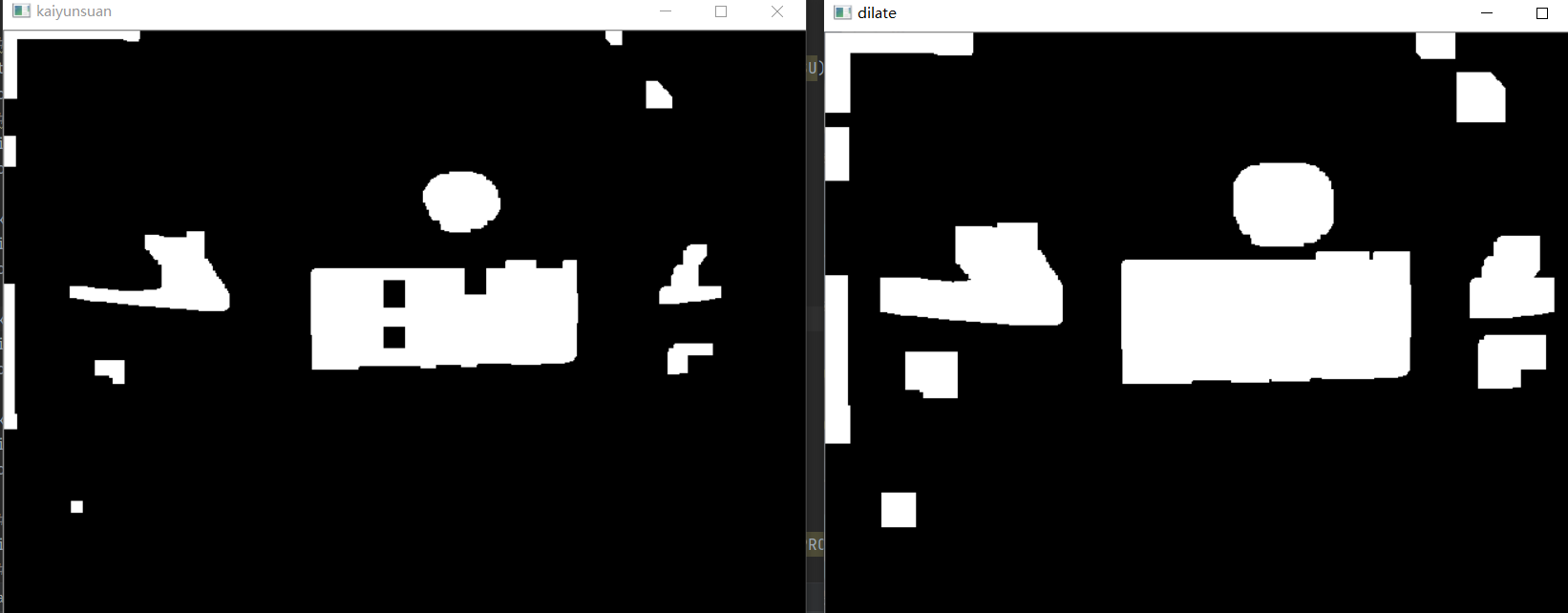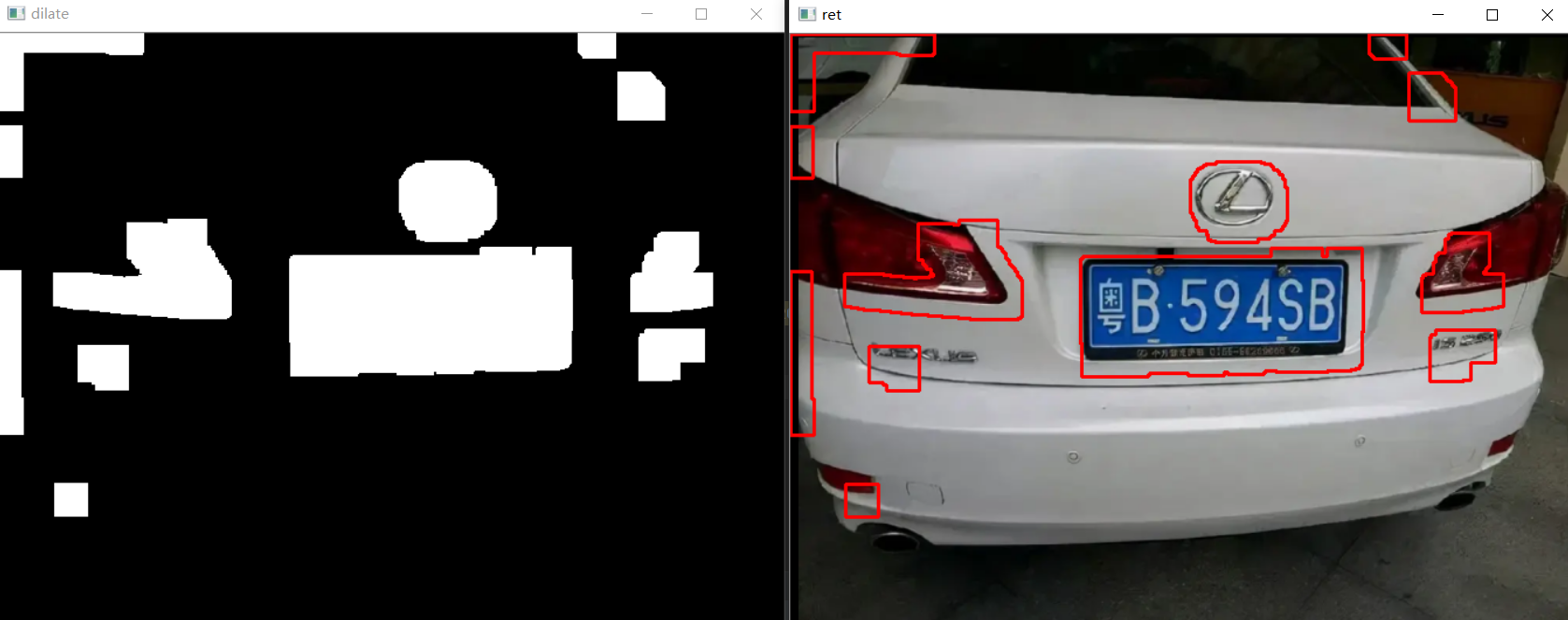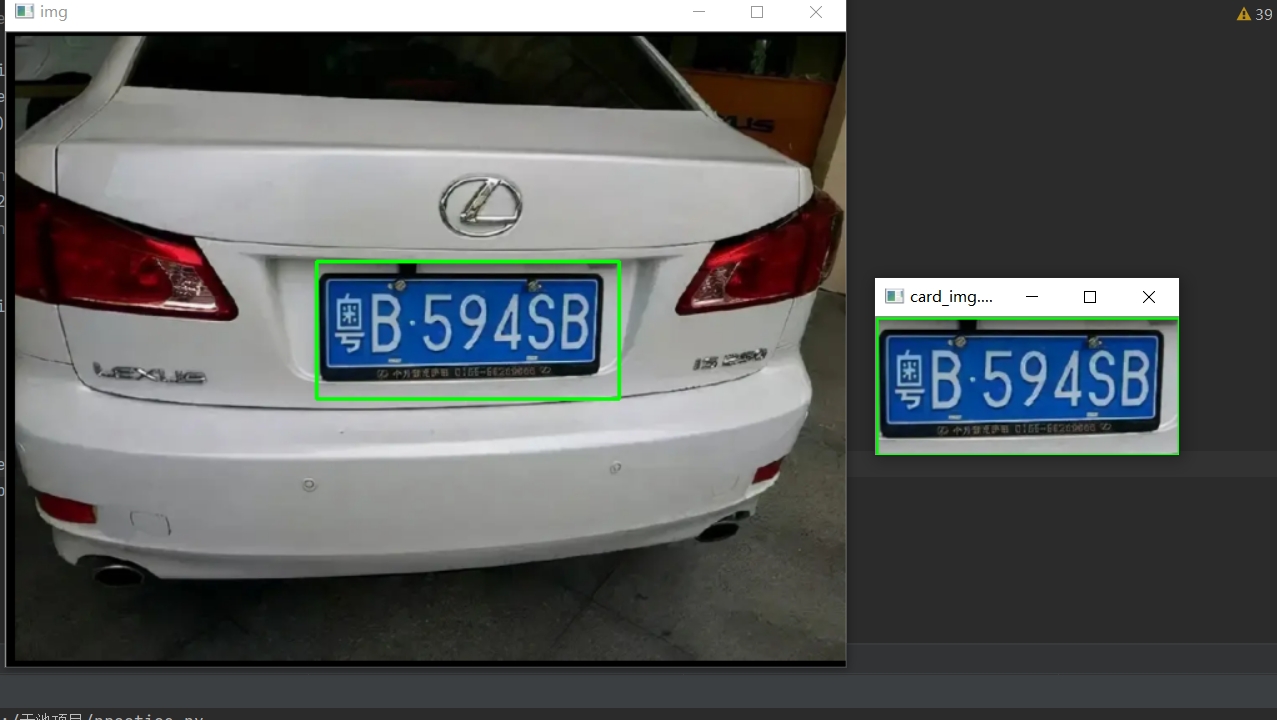``````import cv2
import numpy as np
import matplotlib.pyplot as plt
from scipy import signal
#########该函数能够读取磁盘中的图片文件，默认以彩色图像的方式进行读取

##############这个函数的作用就是来调整图像的尺寸大小，当输入图像尺寸的宽度大于阈值（默认1000），我们会将图像按比例缩小#######
def resize_photo(imgArr,MAX_WIDTH = 1000):
img = imgArr
rows, cols= img.shape[:2]     #获取输入图像的高和宽即第0列和第1列
if cols >  MAX_WIDTH:
change_rate = MAX_WIDTH / cols
img = cv2.resize(img ,( MAX_WIDTH ,int(rows * change_rate) ), interpolation = cv2.INTER_AREA)
return img

# ################高斯平滑###############
#我们首先会对图像水平方向进行卷积，然后再对垂直方向进行卷积，其中sigma代表高斯卷积核的标准差
def gaussBlur(image,sigma,H,W,_boundary = 'fill', _fillvalue = 0):
#水平方向上的高斯卷积核
gaussKenrnel_x = cv2.getGaussianKernel(sigma,W,cv2.CV_64F)
#进行转置
gaussKenrnel_x = np.transpose(gaussKenrnel_x)
#图像矩阵与水平高斯核卷积
gaussBlur_x = signal.convolve2d(image,gaussKenrnel_x,mode='same',boundary=_boundary,fillvalue=_fillvalue)
#构建垂直方向上的卷积核
gaussKenrnel_y = cv2.getGaussianKernel(sigma,H,cv2.CV_64F)
#图像与垂直方向上的高斯核卷积核
gaussBlur_xy = signal.convolve2d(gaussBlur_x,gaussKenrnel_y,mode='same',boundary= _boundary,fillvalue=_fillvalue)
return gaussBlur_xy

def chose_licence_plate(contours, Min_Area=2000):
temp_contours = []
for contour in contours:
if cv2.contourArea(contour) > Min_Area:
temp_contours.append(contour)
car_plate = []
for temp_contour in temp_contours:
rect_tupple = cv2.minAreaRect(temp_contour)
rect_width, rect_height = rect_tupple
if rect_width < rect_height:
rect_width, rect_height = rect_height, rect_width
aspect_ratio = rect_width / rect_height
# 车牌正常情况下宽高比在2 - 5.5之间
if aspect_ratio > 2 and aspect_ratio < 5.5:
car_plate.append(temp_contour)
rect_vertices = cv2.boxPoints(rect_tupple)
rect_vertices = np.int0(rect_vertices)
return car_plate

if len(car_plates)==1:
for car_plate in car_plates:
row_min,col_min = np.min(car_plate[:,0,:],axis=0)
row_max, col_max = np.max(car_plate[:, 0, :], axis=0)
cv2.rectangle(img, (row_min,col_min), (row_max, col_max), (0,255,0), 2)
card_img = img[col_min:col_max,row_min:row_max,:]
cv2.imshow("img", img)
cv2.imwrite( "card_img.png", card_img)
cv2.imshow("card_img.png", card_img)
cv2.waitKey(0)
cv2.destroyAllWindows()
return  "card_img.png"

if __name__ == "__main__":

gray_img = cv2.cvtColor(img, cv2.COLOR_BGR2GRAY)  # 将彩色图片转换为灰色图片,灰色图像更便于后续处理。
rgray_img = resize_photo(gray_img)

# 高斯平滑
blurImage = gaussBlur(gray_img, 5, 400, 400, 'symm')
#对bIurImage进行灰度级显示
blurImage = np.round(blurImage)
blurImage = blurImage.astype(np.uint8)

kernel = np.ones((10, 10), np.uint8)
#开运算
img_opening = cv2.morphologyEx(blurImage, cv2.MORPH_OPEN, kernel)
# cv2.imshow("GaussBlur_gray_img", blurImage)
# cv2.imshow("xingtai_gray_img", img_opening)

#将两幅图像合成为一幅图像
img_opening = cv2.addWeighted(rgray_img, 1, img_opening, -1, 0)
# cv2.imshow("hecheng_gray_img", img_opening)

#阈值分割
t, result_img = cv2.threshold(img_opening, 0, 255, cv2.THRESH_BINARY + cv2.THRESH_OTSU)
# cv2.imshow("yuzhi_gray_img",result_img)
#canny边缘检测
img_edge = cv2.Canny(result_img, 100, 200)
# cv2.imshow("bianyuan_gray_img", img_edge)
#闭运算来填充白色物体内细小黑色空洞的区域并平滑其边界
kernel1 = np.ones((18, 18), np.uint8)
img_edge1 = cv2.morphologyEx(img_edge, cv2.MORPH_CLOSE, kernel1)
# cv2.imshow("biyunsuan", img_edge1)

kernel2 = np.ones((10, 10), np.uint8)
img_edge2 = cv2.morphologyEx(img_edge1, cv2.MORPH_OPEN, kernel2)
# cv2.imshow("kaiyunsuan", img_edge2)

kernel = np.ones((20, 20), np.uint8)
img_dilate = cv2.dilate(img_edge2, kernel)  # 膨胀
cv2.imshow("dilate", img_dilate)  # 显示图片

# #查找图像边缘整体形成的矩形区域, contours是找到的多个轮廓
image, contours, hierarchy = cv2.findContours(img_dilate,cv2.RETR_TREE, cv2.CHAIN_APPROX_NONE)
# cv2.imshow("xunzhao", hierarchy)

draw_img = img.copy()
result = cv2.drawContours(draw_img, contours, -1, (0, 0, 255), 2)
# 画出带有轮廓的原始图片
cv2.imshow('ret',result)
# cv2.waitKey(0)
# cv2.destroyAllWindows()

car_plates = chose_licence_plate(contours)
``````

25 0

pdf(new) 更多>